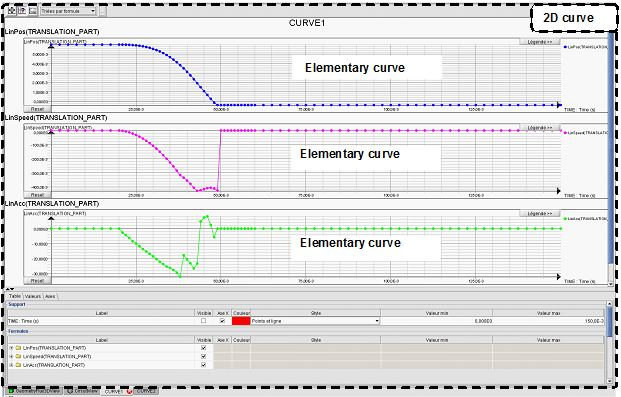## Introduction

The various quantities calculated in Flux can be graphically represented as curves.

One can distinguish two types of curves:

• The curves called « local » with regard to the representation of a local quantity in function of position
• The curves called « temporal » or « parametric » with regard to the representation of a quantity in function of a parameter or of time.

## 2D Curves (Path) and (I/O Parameter)

The two types of curves called in the software 2D Curve (Path) and 2D Curve (I/O Parameter) are presented in the table below.

Type Description
2D Curve (path) curve along a path Graphic representation of one (or more) local quantity(s) in function of the position along a path (previously created)
2D Curve (I/O Parameter) curve in function of an I/O parameter Graphic representation of one (or more) local or global quantity(s) in function of a variable quantity (I/O parameter)

## Operation

The general process comprises the following phases:

Phase Description
1

The user creates a 2D curve defining:

• a name
• a quantity on the abscissa (path, I/O parameter)
• one or more quantities on ordinates (local quantity, I/O parameter)
2

The software creates the 2D curve i.e.:

• calculation of the values corresponding to the elementary curves required by the user
• calculation of the particular quantities associated to these elementary curves (min value, max value, averages, ...)
• open a 2D sheet (specific tab bearing the name of the 2D curve )
• displays the elementary curves in the graphic zone of this 2D sheet
• performs the data storage
3

The user can:

• ... in the 2D sheet:
• visualize the elementary curves (visualization tools)
• perform mathematical operations (tools for derivation, integration, ...)
• edit, export the calculated values
• ... from the data tree:
• edit the particular quantities
• verify the calculation conditions (scenario, ...)

## Vocabulary

Attention, it is necessary to define the vocabulary associated with the objects manipulated in Flux.

In this document, we name:

• curve or elementary curve, a curve (in the usual sense of the term), i.e. the representation of the evolution law of a phenomenon
• 2D Curve*, the entity Flux that regroups one or more elementary curves
Note: * This can be the 2D Curve (path) or the 2D Curve (I/O Parameter) , which are two types of distinct entities in the data tree.

## Example

The notions of 2D curve and of elementary curves are explained in the example below.

The 2D curve called CURVE1 regroups the following elementary curves:

• LinPos(TRANSLATION_PART)
• LinSpeed(TRANSLATION_PART)
• LinAcc(TRANSLATION_PART)